Introduction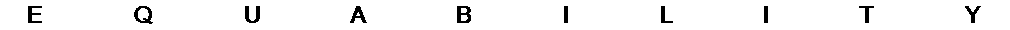INTRODUCTION

Balmoral Software

This page presents terminology, notation, conventions and assumptions regarding the various equable shapes discussed on this site. The mathematics included within are at about the high-school or early undergraduate level.

### Two-dimensional shapes

A planar shape is said to be equable if its area A and perimeter P are numerically equal. A simple example is a square with side length 4, which has its perimeter and area both equal to 16. We may intuitively expect equable shapes to have at least some of their dimensions relatively small. This is because enlarging the sides of a convex polygon by a factor of k > 1 increases the perimeter by the same factor, but the area is increased by the larger factor k2.

In these pages, we are interested in equable convex plane figures that have integer area and sides. Regular n-sided polygons are excluded because their areas

A = (1/4)ns2 cot(π/n), n ≥ 3
are irrational, except for the square n = 4, which can be treated as a special case of the quadrilateral.

### Area- and perimeter-dominant polygons

Results are also presented for integer-sided triangles and quadrilaterals that are not equable, and may not have integer area. An area-dominant polygon is defined as one for which A > P, while the term perimeter-dominant is used for those with A < P. The former category is important in finding polygonal bases for equable right prisms, and the relatively less common second category will be used in the analysis of certain triangles, quadrilaterals and pentagons.

### Three-dimensional solids

In three dimensions, an equable solid has numerically equal volume V and total surface area S. We will focus on equable convex solids with volumes that are integers, or integer multiples of π. The individual surface areas defined by edges will also be integers or integer multiples of π, analogous to the sides of equable two-dimensional figures. In cases where these restrictions fail to limit the outcomes to a finite number of equable solids, we may further assume that a dimension or its square is an integer or rational number. Regular Platonic, Archimedean, Catalan and Johnson solids (except for the cube) are excluded because their volumes and/or surface areas are irrational.

Equability, or the condition of being equable, is clearly an abstraction only. In any real-world scenario, the fallacy of equating units of length and area, or of area and volume, would lead to conclusions that are unscalable.

### Cuboids

A cuboid with rational dimensions has the property that its volume automatically satisfies the integer convention if its six individual surface areas do:
The volume of a rectangular prism with rational dimensions is an integer if all of its faces have integer areas.
Proof. Equivalently, for positive rational numbers x, y and z, if xy, xz and yz all are integers, then xyz is an integer. Let
xy = k
xz = l
yz = m,
for positive integers k, l and m. Then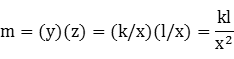Let x = p/q for positive integers p and q. We can write the preceding equation as
mx2 - kl = 0
This is a quadratic equation in x with integer coefficients, so by the Rational Root Theorem, p divides kl:for some positive integer n1. Define the integer n2 as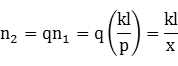Then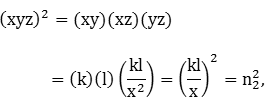so xyz is an integer, QED.

### Prisms

Some closed right prisms are equable. A right prism with base perimeter P and integer base area A has a lateral surface area equal to the product of P and the prism height h, and the equability requirement is
 V = hA = 2A + hP = S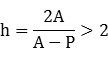Therefore, the base shape of an equable right prism must be area-dominant, and thus cannot itself be equable. The prism height h = V/A is rational. It follows from  that the perimeter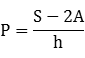is also rational.

Suppose there exists an equable solution with integer base area A, rational base perimeter P and rational height h. If we multiply all sides of the base by an integer n greater than 1, the new perimeter and area are

P' = nP ∈ ℚ

A' = n2A ∈ ℤ,

and the corresponding height to preserve equability is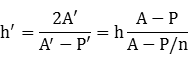Since the denominator is positive, another equable solution is generated, so there are infinitely-many equable prisms with integer base area, rational base perimeter and rational height.

### Integer prism dimensions

Henceforth, we will restrict prism dimensions to be integers to discover the more distinctive equable cases. If the rational number t represents the ratio of base area to perimeter, an equable prism has the property that
t = A/P = h/(h - 2),
or equivalently,
h = 2t/(t - 1) = 2 + 2/(t - 1)
When h is an integer, we have the following results:
tSolutions for integer h
(0,1]none
(1,2)h ≥ 5
24
(2,3)none
33
(3,∞)none
The following relationships also hold:
ht⌊4t2⌊4t2⌋ - 4t2 + 1
33361
42161
55/3118/9
63/291
7 or 84/3 or 7/574/25 or 8/9
9 or 105/4 or 9/7619/49 or 3/4
11 to 189/8 to 11/952/81 to 15/16
≥ 191 < t ≤ 19/174≥ 1/289

where ⌊⌋ is the floor function.

We conclude that
1/289 ≤ ⌊4t2⌋ - 4t2 + 1 ≤ 1
for any values of interest for t.

### Summary

 2 dimensions 3 dimensions Equable requirement: Area = Perimeter Volume = Surface Area Integer requirement: Area & dimensions Volume & surface areasDimensions (prisms)
Many solutions require solving Diophantine equations.

The rest of this paper presents definitions and miscellaneous results that will be used in the analysis of specific equable shapes.

### Division by a product

Let a, b and c be integers. If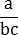∈ ℤ,
then b | a and c | a.

Proof. Clearly b | bc, and by assumption, bc | a. Then by the transitive property of divisibility, b | a. Similarly, c | bc, so c | a.

### Heronian polygons

Various pages on this site discuss Heronian triangles and quadrilaterals. For convenience, we use the convention that a Heronian polygon is convex and has integer sides and area. Some authors use the definition of a Heronian polygon as one with rational side lengths and rational area, but any such polygon can be converted into one of the present definition by multiplying by the least common multiple of the sides and area. We do not require that the diagonals of a Heronian quadrilateral also be integers.

### Generalized polygon inequality

The generalized polygon inequality is satisfied for a polygon with sides a1,a2,... when
max{a1,a2,...} < s,
where s = (a1 + a2 + ...)/2 is the semiperimeter of the polygon. It follows that s - ai is strictly positive for all i.

Proof. When the inequality is satisfied, we have

ai < s
for all i. Then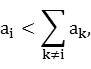so side ai is less than the sum of all the other sides, which is the generalized polygon inequality.

### Area of Heronian isosceles triangle

The area of a Heronian isosceles triangle is a multiple of 3.
Proof. Let the integer legs and base of an isosceles triangle be a and b, respectively. The area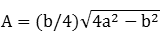of the triangle is an integer. Then
(4A)2 + (b2)2 = (2ab)2,
so (4A,b2,2ab) constitute a Pythagorean triple. Since 3 and 16 are relatively prime, it suffices to show that 16A2 is a multiple of 3. By the Lemma below, 3 divides 4A and/or 3 divides b2. In the former case, we are done. In the latter case, 3 divides b, so 3 divides 2ab and the right side above is a multiple of 3. For the left side to also be a multiple of 3, 16A2 must be a multiple of 3, Q.E.D.

Lemma. At least one of the two smallest members of a Pythagorean triple is a multiple of 3.

Proof. Let the integer elements of a Pythagorean triple be a, b and c, where

a2 + b2 = c2
A square mod 3 cannot be 2, so we have
(a2 + b2) mod 3 = c2 mod 3 ∈ {0,1}
If a2 mod 3 = 1 and b2 mod 3 = 1, then the left side is 2, a contradiction. Therefore, at least one of {a2,b2} is a multiple of 3, and the conclusion follows.

### Pythagorean fraction limits

For a Pythagorean triple of positive integers a,b,c with a2 + b2 = c2, we have
 b2/a > 2 for all a and b  b2/a ≥ 16/3 = 5.333... if a < b 
Proof. We can write
c2 = a2 + b2 > a2

c > a

c ≥ a + 1

c2 ≥ (a + 1)2 > a2 + 2a

b2 = c2 - a2 > 2a,

which proves .

The Pythagorean triple with the shortest leg is 3,4,5, so a ≥ 3. If b > a, then

b ≥ a + 1

b2 ≥ (a + 1)2

= (a - 3)(a - 1/3) + 16a/3

≥ (3 - 3)(3 - 1/3) + 16a/3 = 16a/3,

which proves .

### Integer squares of rational numbers

If x is rational and x2 is an integer, then x is an integer.
Proof. If x2 is an integer, it can be written as
x2 = m, for some integer m,
or as a monic polynomial in x with integer coefficients:
x2 - m = 0.
By the integral root theorem, x is an integer. The converse is trivially true.

### Pell recursion

A Pell equation is of the form
 x2 - Dy2 = 1 
where x and y are positive integers and D ≥ 2 is a positive nonsquare integer. Solutions to  for a particular value of D are an infinite integer sequence {xn,yn} that is generated recursively by a second-order linear difference equation with initial conditions. The closed-form solutions to  are well known: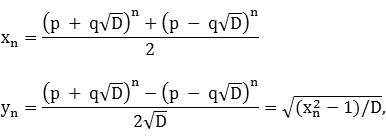where (p,q) is an initial solution for (x,y) in  that depends on D. The initial solution is generally selected with positive p and q, and so xn → ∞ as n → ∞ since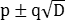are both positive. In practice, the initial solution is found by inspection, table lookup, or a solution algorithm such as the Chakravala method. Let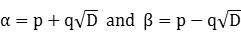Note that αβ = p2 - q2D = 1 since (p,q) is a solution to . Applying Newton's identity, we have
αn ± βn

= (α + β)(αn-1 ± βn-1) - αn-1β ∓ αβn-1

= (α + β)(αn-1 ± βn-1) - αβ(αn-2 ± βn-2)

= 2p(αn-1 ± βn-1) - (αn-2 ± βn-2)

The sequence 2xn is of this form (with + sign), and
2x0 = α0 + β0 = 2

2x1 = α + β = 2p,

so its recursive solution is the second-order linear difference equation
 xn = 2pxn-1 - xn-2, x0 = 1, x1 = p 
The sequence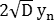is also of this form (with - sign), andso its recursive solution is the same second-order linear difference equation, but with different initial conditions:
 yn = 2pyn-1 - yn-2, y0 = 0, y1 = q 
Consider the modified Pell equation
(a1xn + b1)2 - D(a2yn + b2)2 = 1
for given constants a1, b1, a2, b2, D and associated parameter p, with a1, a2 ≠ 0. The second-order linear difference equations for xn and yn can be found as follows:

By ,

a1xn + b1 = 2p(a1xn-1 + b1) - (a1xn-2 + b1)

xn = 2pxn-1 - xn-2 + 2b1(p - 1)/a1,

and analogously,
yn = 2pyn-1 - yn-2 + 2b2(p - 1)/a2,
with suitably-chosen initial conditions.

We can write  as

(xn2 - 1)/yn2 = D
so
xn/yn → D as n → ∞
and### Non-squarefree Pell Equations

A non-squarefree coefficient in a Pell equation produces a subset of the solution sequence from the corresponding primitive Pell equation. If (xn,yn) is a solution sequence for
x2 - Dy2 = 1
then for a given positive integer m,
 x'2 - m2Dy'2 = 1 
has a solution sequence (x'n,y'n) = (xn,yn/m), where {x'n} ⊆ {xn}.

Proof. Let

 k = smallest n such that m | yn 
Then
xk2 = (yk/m)2m2D + 1
and (x'n,y'n) = (xnk,ynk/m) is a solution to the modified Pell equation . The modified problem has solutions appearing every kth term of (xn,yn).

For example, take D = 6 and m = 11. Then we can see that k = 3 by examining the solution sequences for D and m2D:

```                   D =    6                                         m2D =  726
p =    5                                         p =    485
q =    2                                         q =     18
----------------------------------------------   ----------------------------------------------
n                     x                     y                     x                     y    n

0                     1                     0                     1                     0    0
1                     5                     2
2                    49                    20
3                   485                   198                   485                    18    3
4                  4801                  1960
5                 47525                 19402
6                470449                192060                470449                 17460    6
7               4656965               1901198
8              46099201              18819920
9             456335045             186298002             456335045              16936182    9
10            4517251249            1844160100
11           44716177445           18255302998
12          442644523201          180708869880          442644523201           16428079080   12
13         4381729054565         1788833395802
14        43374646022449        17707625088140
15       429364731169925       175287417485598       429364731169925        15935219771418   15
16      4250272665676801      1735166549767840
17     42073361925598085     17176378080192802
18    416483346590304049    170028614252160180    416483346590304049     15457146750196380   18
```
A table of values for k is easily created using  and , as described in the Python code for OEIS sequence A298210:
```                         Table of k values

m:  2  3  4  5  6  7  8  9 10 11 12 13 14 15 16 17 18 19 20     OEIS
D   p   q
2   3   2  1  2  2  3  2  3  4  6  3  6  2  7  3  6  8  4  6 10  6	A298210
3   2   1  2  3  2  3  6  4  4  9  6  5  6  6  4  3  8  9 18  5  6	A298211
5   9   4  1  2  1  5  2  4  2  2  5  5  2  7  4 10  4  3  2  3  5	A298212
6   5   2  1  3  2  2  3  4  4  3  2  3  6  7  4  6  8  9  3  9  2
7   8   3  2  1  2  3  2  7  2  3  6  6  2  7 14  3  2  3  6  9  6
8   3   1  2  2  4  3  2  3  8  6  6  6  4  7  6  6 16  4  6 10 12
10  19   6  1  1  2  5  1  4  4  3  5  6  2  3  4  5  8  9  3  2 10
11  10   3  2  1  2  2  2  3  4  3  2 11  2  7  6  2  8  9  6  3  2
12   7   2  1  3  2  3  3  2  4  9  3  5  6  3  2  3  8  9  9  5  6
13 649 180  1  1  1  1  1  4  2  1  1  2  1 10  4  1  4  4  1 38  1
14  15   4  1  2  1  2  2  7  2  6  2  5  2  6  7  2  4  9  6 10  2
15   4   1  2  3  2  5  6  3  2  3 10  5  6  7  6 15  4  8  6 10 10
17  33   8  1  2  1  3  2  4  1  6  3  2  2  3  4  6  2 40  6  9  3
18  17   4  1  3  1  3  3  3  2  9  3  3  3  7  3  3  4  2  9  5  3
19 170  39  2  1  2  2  2  4  4  3  2  3  2  1  4  2  8  2  6 21  2
20   9   2  1  2  2  5  2  4  4  2  5  5  2  7  4 10  8  3  2  3 10
```

### Isoperimetric inequality

The isoperimetric inequality can be used to determine the minimum areas and volumes of two- and three-dimensional equable shapes.

In the case of a planar shape, we have

P ≥ 2A1/2(area of unit circle)1/2 = 2(Aπ)1/2

P2 ≥ 4Aπ

Since P = A for equability, we have
A ≥ 4π = 12.5664
This minimum is reached for the equable circle, and the areas of all other equable two-dimensional shapes exceed it.

In the case of a three-dimensional shape, we have

S ≥ 3V2/3(volume of unit sphere)1/3 = 3V2/3(4π/3)1/3

S3 ≥ 27V24π/3 = 36V2π

Since S = V for equability, we have
V ≥ 36π = 113.0973
This minimum is reached for the equable sphere, and the volumes of all other equable three-dimensional shapes exceed it.

### Solids of revolution

An equable planar shape may give rise to a convex equable solid by rotating it around one of its straight edges. Of all the equable two-dimensional shapes on these pages, the following five produce equable convex solids of revolution:
 A = P Axis of rotation V = S Equable shape Equable solid of revolution 6 x 8 right triangle 24 Long leg Right circular cone with r = 6, h = 8 96π 4 x 4 square 16 Any side Cylinder with r = h = 4 64π 6 x 3 rectangle 18 Short side Cylinder with r = 6, h = 3 108π 6 x 3 rectangle 18 Long side Cylinder with r = 3, h = 6 54π (6,5,5,1) rectangular pentagon 18 Base Conical bi-frustum with r1 = 1, r2 = 5, h = 3 62π
An example for which the solid of revolution is not convex is a torus created by rotating an equable circle of radius 2 around a straight line outside of the circle. This torus is itself equable, regardless of the distance R of the center of the circle from the axis of rotation:
V = 2π2Rr2 = 4π2Rr = S

r = 2

### Reference

W. Sierpinski, Elementary Theory of Numbers, Polish Scientific Publishers, Warsaw, Poland, 1988, p. 11.